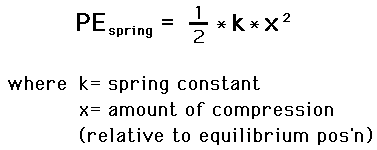# A ball with a mass of 70 g is projected vertically by a spring loaded contraption. The spring in the contraption has a spring constant of 49 (kg)/s^2 and was compressed by 7/6 m when the ball was released. How high will the ball go?

A ball with a mass of 70 g is projected vertically by a spring loaded contraption. The spring in the contraption has a spring constant of 49 (kg)/s^2 and was compressed by 7/6 m when the ball was released. How high will the ball go?

ReportAnswer 1

The height is $= 48.61 m$

#### Explanation:The spring constant is $k = 49 k g {s}^{-} 2$

The compression is $x = \frac{7}{6} m$

The potential energy in the spring is

$P E = \frac{1}{2} k {x}^{2} = \frac{1}{2} \cdot 49 \cdot {\left(\frac{7}{6}\right)}^{2} = 33.35 J$

This potential energy will be converted to kinetic energy when the spring is released and to potential energy of the ball

$K {E}_{b a l l} = \frac{1}{2} m {u}^{2}$

Let the height of the ball be $= \left(h\right) m$

Then ,

The potential energy of the ball is $P {E}_{b a l l} = m g h$

The mass of the ball is $= 0.070 k g$

The acceleration due to gravity is $g = 9.8 m {s}^{-} 2$

$P {E}_{b a l l} = 33.35 = 0.070 \cdot 9.8 \cdot h$

The height is $h = 33.35 \cdot \frac{1}{0.070 \cdot 9.8}$

$= 48.61 m$

##### Add Answer of: A ball with a mass of 70 g is projected vertically by a spring loaded contraption. The spring in the contraption has a spring constant of 49 (kg)/s^2 and was compressed by 7/6 m when the ball was released. How high will the ball go?
Similar Homework Help Questions